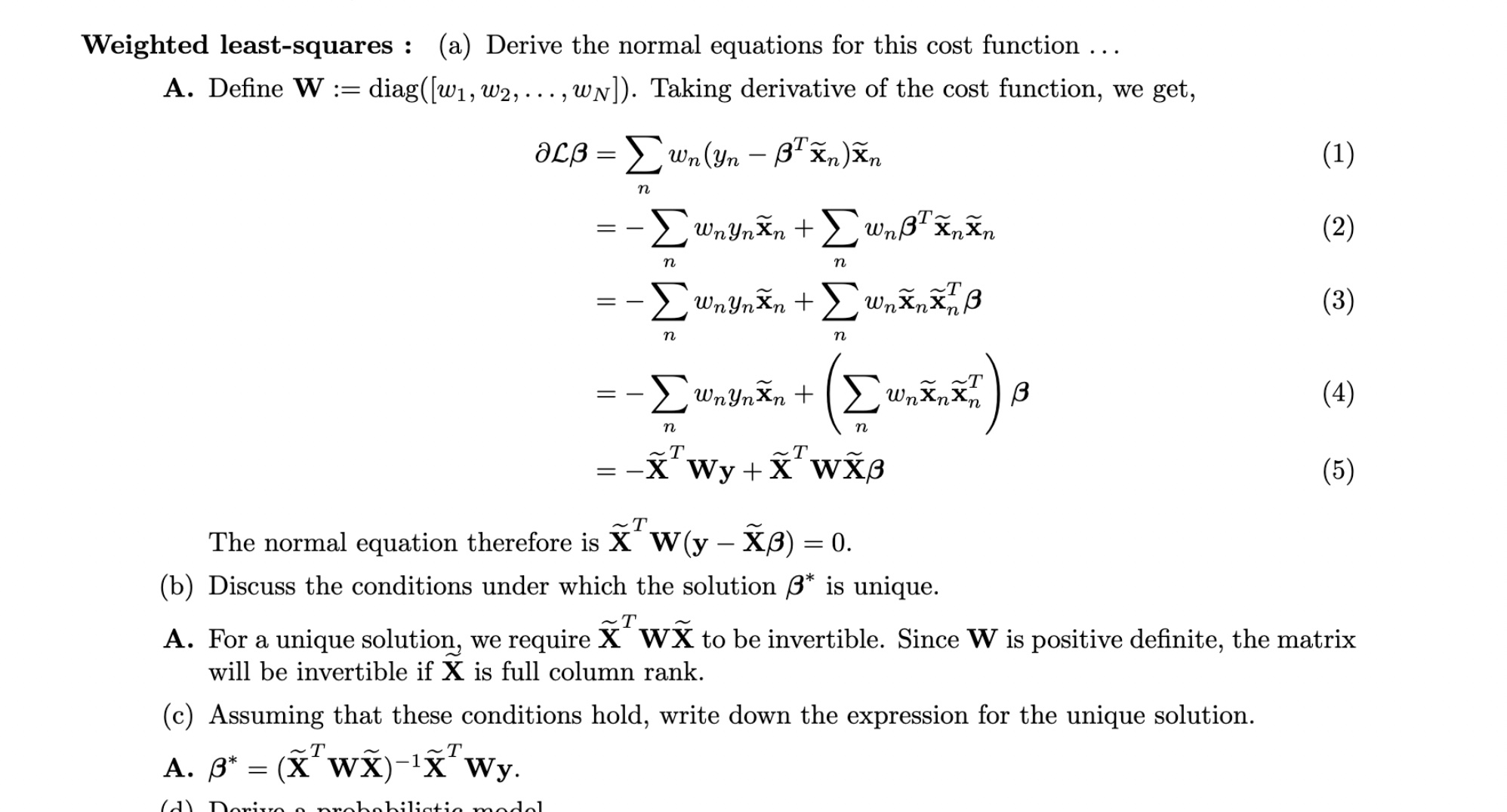### Mock exam 2014 invertible matrix conditions

Hello,

For the question (b) below, I agree that W is positive definite since it's a diagonal matrix, so the eigen values are the values on the diagonal, i.e. the wi which are all > 0. But then, why X_TWX is invertible if X is full column rank? Where does that condition come from?Top comment

The proof goes in the same as to why $$X^\top X$$ would be invertible if X is full column rank.

Basically $$null(X^\top X)= null(X)$$, to show this pick $$w\in null(X^\top X)$$, this means $$w^\top X^\top X w=0$$, this is the same as $$\Vert Xw\Vert^2=0$$ which means $$Xw=0$$ i.e. $$w\in null(X)$$ , the other implication is straightforward.
So now if $$X$$ is full column rank i.e. the columns of $$X$$ are linearly independent, then $$rank(X)=D$$, using the rank equality $$rank(X) + dim null(X)=D$$ we get that $$dim null(X)=0$$ which means dim $$null(X^\top X)=0$$ which means $$rank(X^\top X)=D$$ and that $$X^\top X$$ is invertible.

Now with the matrix (symmetric) positif definite matrix W added,we still have $$null(X^\top W X)= null(X)$$, now if $$w\in null(X^\top W X$$) then $$w^\top X^\top W X w=0$$ which means $$\Vert W^{1/2}Xw\Vert^2=0$$ which implies $$W^{1/2}Xw=0$$ implies $$Xw=0$$ which concludes the proof.

Hi, where can you find these older mock exams? They are not on the course website?

@tomas2 said:
Hi, where can you find these older mock exams? They are not on the course website?

Yes they are in the github in the folder mock midterm exams :)

Top comment

The proof goes in the same as to why $$X^\top X$$ would be invertible if X is full column rank.

Basically $$null(X^\top X)= null(X)$$, to show this pick $$w\in null(X^\top X)$$, this means $$w^\top X^\top X w=0$$, this is the same as $$\Vert Xw\Vert^2=0$$ which means $$Xw=0$$ i.e. $$w\in null(X)$$ , the other implication is straightforward.
So now if $$X$$ is full column rank i.e. the columns of $$X$$ are linearly independent, then $$rank(X)=D$$, using the rank equality $$rank(X) + dim null(X)=D$$ we get that $$dim null(X)=0$$ which means dim $$null(X^\top X)=0$$ which means $$rank(X^\top X)=D$$ and that $$X^\top X$$ is invertible.

Now with the matrix (symmetric) positif definite matrix W added,we still have $$null(X^\top W X)= null(X)$$, now if $$w\in null(X^\top W X$$) then $$w^\top X^\top W X w=0$$ which means $$\Vert W^{1/2}Xw\Vert^2=0$$ which implies $$W^{1/2}Xw=0$$ implies $$Xw=0$$ which concludes the proof.